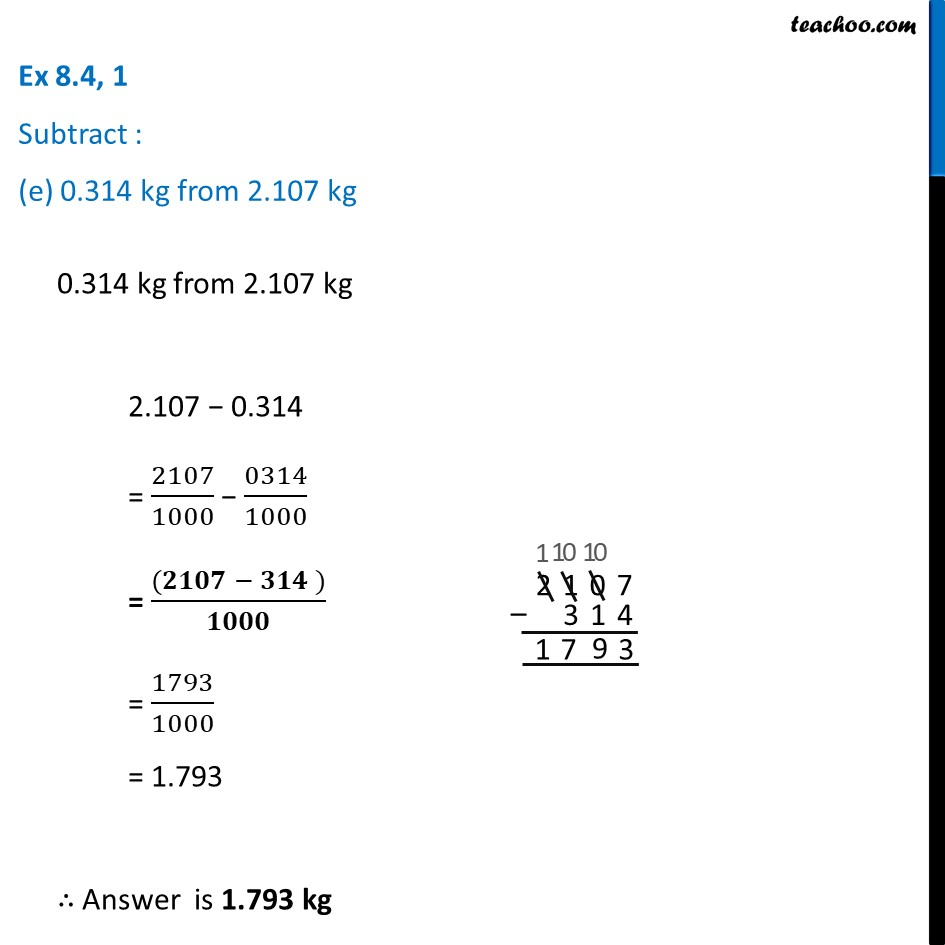Ex 8.4

Chapter 8 Class 6 Decimals
Serial order wiseLearn in your speed, with individual attention - Teachoo Maths 1-on-1 Class

### Transcript

Ex 8.4, 1 Subtract : (e) 0.314 kg from 2.107 kg0.314 kg from 2.107 kg 2.107 − 0.314 = 2107/1000 − 0314/1000 = ((𝟐𝟏𝟎𝟕 − 𝟑𝟏𝟒 ))/𝟏𝟎𝟎𝟎 = 1793/1000 = 1.793 ∴ Answer is 1.793 kg